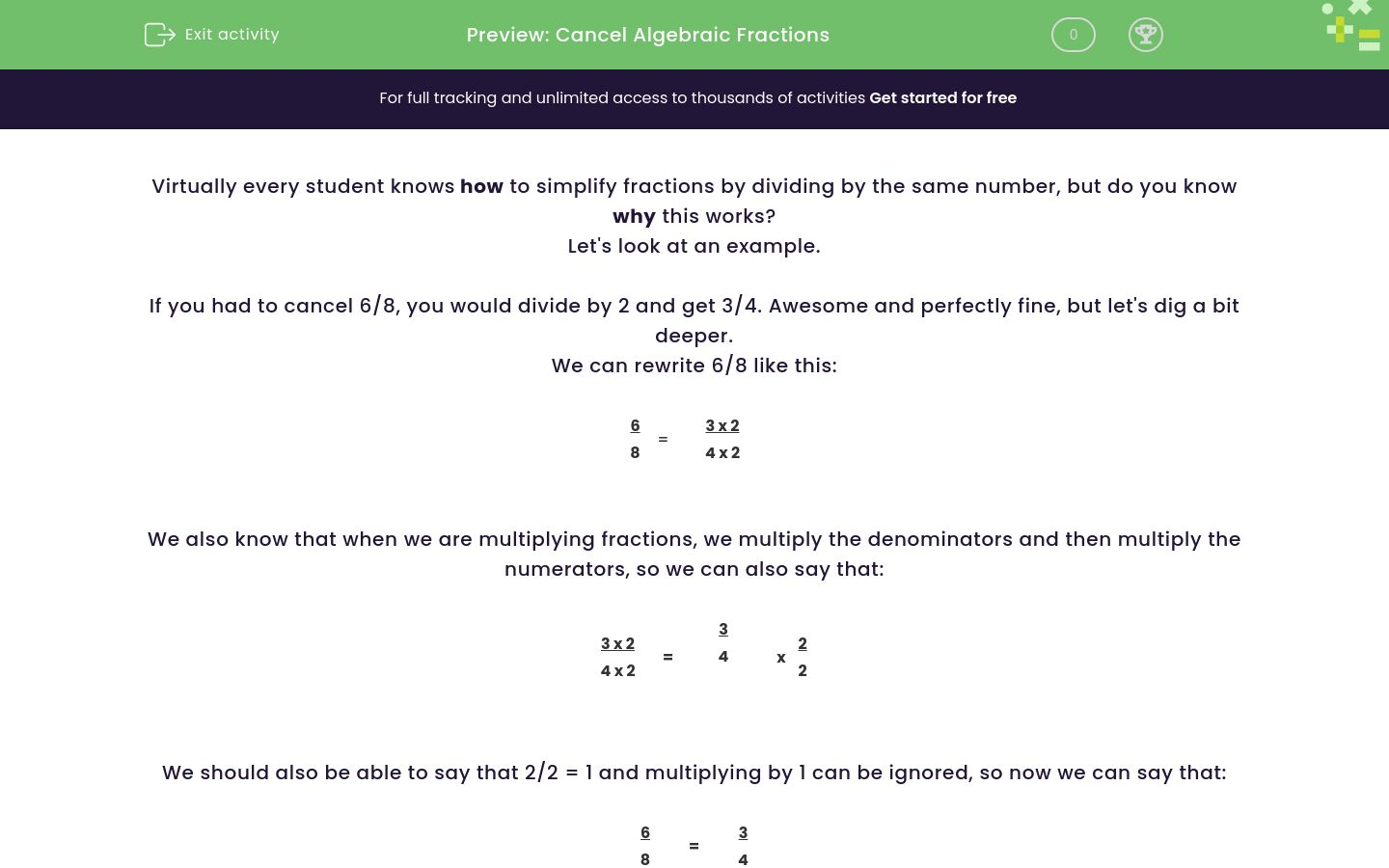# Cancel Algebraic Fractions

In this worksheet, students will practise simplifying algebraic fractions by factorising.Key stage:  KS 4

GCSE Subjects:   Maths

GCSE Boards:   AQA, Eduqas, OCR, Pearson Edexcel,

Curriculum topic:   Algebra

Curriculum subtopic:   Notation, Vocabulary and Manipulation Algebraic Expressions

Difficulty level:#### Worksheet Overview

Virtually every student knows how to simplify fractions by dividing by the same number, but do you know why this works?

Let's look at an example.

If you had to cancel 6/8, you would divide by 2 and get 3/4. Awesome and perfectly fine, but let's dig a bit deeper.

We can rewrite 6/8 like this:

 6 8
=
 3 x 2 4 x 2

We also know that when we are multiplying fractions, we multiply the denominators and then multiply the numerators, so we can also say that:

 3 x 2 4 x 2
=
 3 4

x
 2 2

We should also be able to say that 2/2 = 1 and multiplying by 1 can be ignored, so now we can say that:

 6 8
=
 3 4

Why is this important?

You would never be asked to show all this working if you were cancelling down a fraction like 6/8 ,so why is it important?

It's because the technique shown which is called cancelling by factorisation, is the only way to cancel fractions that involve algebra.

How to cancel by factorisation

Whenever you see a fraction involving algebra, remember that you have to factorise it first.

Example 1:

Simplify the following fraction:

 15x2 + 5x 30x + 10

If we look at each line individually:

15x2 + 5x can be factorised to 5x(3x + 1)

30x + 10 can be factorised to 10(3x + 1)

So we can rewrite our fraction as:

 15x2 + 5x 30x + 10
=
 5x(3x + 1) 10(3x + 1)

Anything that is the same on the top and bottom can now be cancelled.

 5x (3x + 1) 210 (3x + 1)
=
 x 2

Example 2:

Simplify the following fraction:

 2x2- 2x - 3 3x2- 5x -12

If we look at each line individually:

2x2- 2x - 3 can be factorised to (2x + 1)(x - 3)

3x2- 5x -12 can be factorised to (3x + 4)(x - 3)

So we can rewrite our fraction as:

 2x2- 2x - 3 3x2- 5x -12
=
 (2x + 1)(x - 3) (3x + 4)(x - 3)

Anything that is the same on the top and bottom can now be cancelled.

 (2x + 1)(x - 3) (3x + 4)(x - 3)
=
 2x + 1 3x + 4

Let's try some questions now.

### What is EdPlace?

We're your National Curriculum aligned online education content provider helping each child succeed in English, maths and science from year 1 to GCSE. With an EdPlace account you’ll be able to track and measure progress, helping each child achieve their best. We build confidence and attainment by personalising each child’s learning at a level that suits them.

Get started